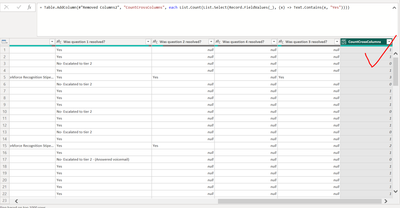cancel
Showing results for
Did you mean:Helper I

## Count specific text values across Several Columns

Hi Friends,

I have a question about creating a column in Power BI editor table that counts the number of a specific text value across several columns.   See the screenshot below, I would like to count how many of the 4 columns ("Was question 1,2,3,4 resolved"?) have a vlaue of "Yes".There is an similar question asked in this forum, and the solution is to use the "List.Count(List.Select(Record.FieldValues(_), (x) => Text.Contains(x, "Yes")))" formulae. I tried this in my case, but what I got is just Errors, although the no syntax errors are detected, as shown in the Screenshot below.I don't know why the formulae does not work in my case, or why it worked in the original case. Is there anyway I can get the count of the values in one step? Thanks.

1 ACCEPTED SOLUTIONHelper I

Thanks, Hotchilli,

Here is the screenshot for the error message. It says " ... cannot convert value 11 to Type text". It is the first ID value, which is a numeric field. I have several numeric field, I think the problem is with the numeric fields.So I excluded all numeric fields from the table, and do the formulae again. This time it works perfect.

See screenshot below.So it seems the formula only works for data with no numeric fields. So if I want to keep my numeric fields in the table, what revision should be made to the formula so it will ignore the numeric fields?

Thanks,

2 REPLIES 2Super User

You click in the Error cell (but not on the word itself)Helper I

Thanks, Hotchilli,

Here is the screenshot for the error message. It says " ... cannot convert value 11 to Type text". It is the first ID value, which is a numeric field. I have several numeric field, I think the problem is with the numeric fields.So I excluded all numeric fields from the table, and do the formulae again. This time it works perfect.

See screenshot below.So it seems the formula only works for data with no numeric fields. So if I want to keep my numeric fields in the table, what revision should be made to the formula so it will ignore the numeric fields?

Thanks,